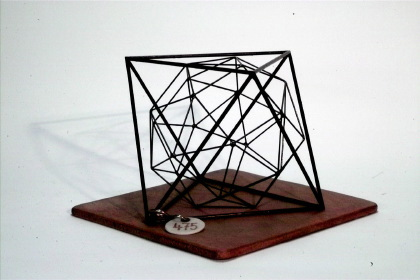Göttingen Collection of Mathematical Models and Instruments

# Octahedron with inscribed snub cube

### Model 475Category: B II 180

### Description

Octahedron with inscribed (6+8+24)-plane polygon with 24 vertices (green), polar figure of the pentagon icositetrahedron.

OctahedronThe octahedron is one of the five Platonic solids, see also model 702.

Inscribed (6+8+24)-plane polygon with 24 vertices The solid inscribed in the octahedron is a snub cube. The snub cube has

6 squares + 8 triangles + 24 triangles = 38 faces.

It has 24 vertices and 60 edges. At each vertice four triangles and one squares are meeting (3,3,3,3,4).
There is also a mirror image of the snub cube.

The snub cube is one of 13 Archimedean solids, see also 482.

Pentagonal icositetrahedron The snub cube is polar (dual) to the pentagonal icositetrahedron. To create this new solid a sphere is inscribed in the snub cube such that the sphere touches each of the faces in exactly one point. These points of contact create the vertices of the dual solid. By connecting these 38 vertices 24 pentagons are formed. These pentagons are the faces of the pentagonal icositetrahedron. The number of edges remains the same during the transformation into the dual solid, while the number of vertices and faces is exchanged.

There are 11 Archimedean solids in the collection.

 472 Truncated tetrahedron inscribed in a tetrahedron 473 Truncated octahedron inscribed in an octahedron 474485 Cuboctahedron 474 inscribed in an octahedron485 inscribed in a cube 475 Truncated cube inscribed in an octahedron 476 Rhombicuboctahedron inscribed in a cube 478 Truncated icosahedron inscribed in an icosahedron 479 truncated dodecahedron inscribed in a dodecahedron 480481 Icosidodecahedron 480 inscribed in a dodecahedron481 inscribed in an icosahedron 482 Rhombicosidodecahedron 483 Snub dodecahedron 484 Truncated cube inscribed in a cube

The truncated icosidodecahedron and the lost truncated cuboctahedron are not included in the collection.

Showcase of this model is Case number 21

### References

Heesch, H.. Comm.Math.Helv, 6, n==5, Case 2a.

Hess, E.(1883). Kugelteilung, Teubner, mit Figuren, §42, fig. 24.